• +91 9971497814
• info@interviewmaterial.com

# Triangles EX-6.4 Interview Questions Answers

### Related Subjects

Question 1 : Let ∆ABC ~ ∆DEF and their areas be, respectively, 64 cm2 and 121 cm2. If EF = 15.4 cm, find BC.

Answer 1 :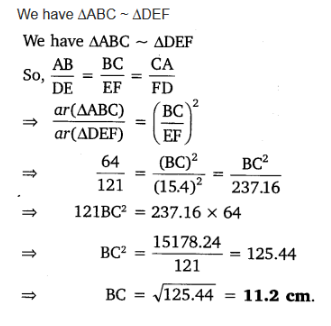Question 2 : Diagonals of a trapezium ABCD with AB || DC intersect each other at the point O. If AB = 2 CD, find the ratio of the areas of triangles AOB and COD.

Answer 2 :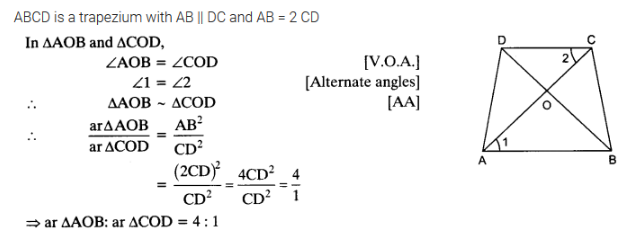Question 3 :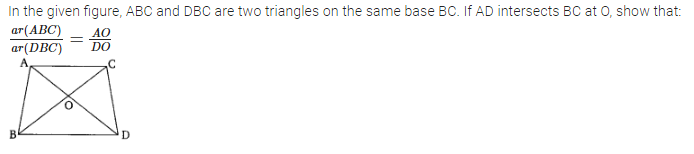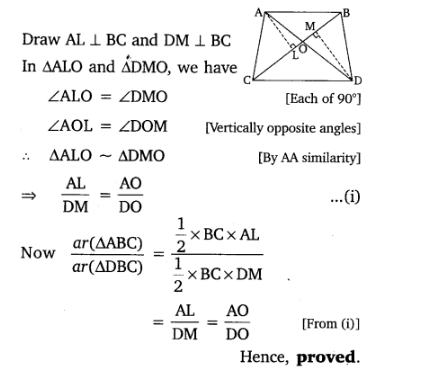Question 4 : If the areas of two similar triangles are equal, prove that they are congruent.

Answer 4 :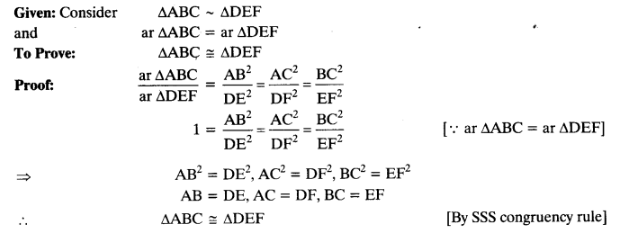Question 5 :

D, E and F are respectivelythe mid-points of sides AB, BC and CA of ΔABC. Find the ratio of the area ofΔDEF and ΔABC.

Answer 5 :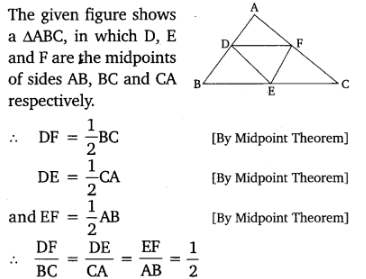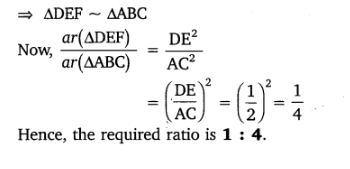Question 6 : Prove that the ratio of the areas of two similar triangles is equal to the square of the ratio of their corresponding medians.

Answer 6 :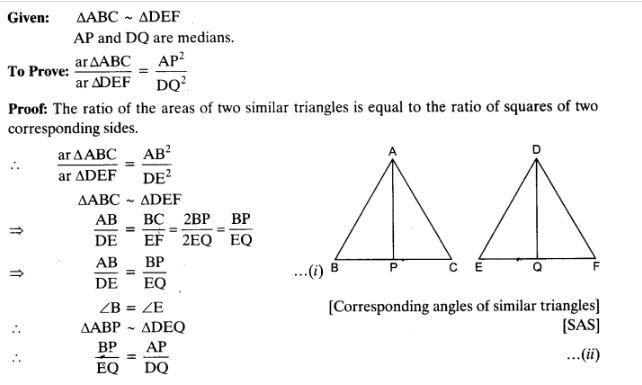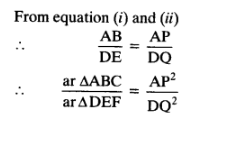Question 7 : Prove that the area of an equilateral triangle described on one side of a square is equal to half the area of the equilateral triangle described on one of its diagonals.

Answer 7 :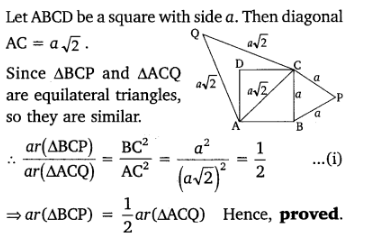Question 8 :
Tick the correct answer and justify

(i) ABC and BDE are two equilateral triangles such that D is the mid-point of BC. Ratio of the areas of triangles ABC and BDE is
(a) 2 :1            (b) 1:2         (c) 4 :1              (d) 1:4

(ii) Sides of two similar triangles are in the ratio 4 : 9. Areas of these triangles are in the ratio
(a) 2 : 3            (b) 4 : 9        (c) 81 : 16         (d) 16 : 81

Solution
(i)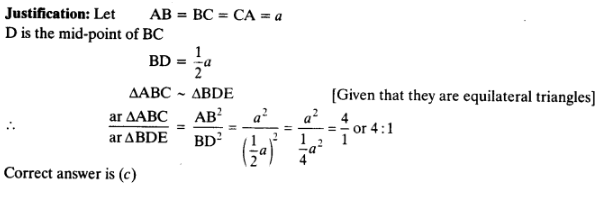Solution
(ii)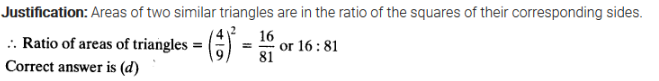Todays Deals### Triangles EX-6.4 Contributorskrishan

Name:
Email:

# Latest News# 9000 interview questions in different categories